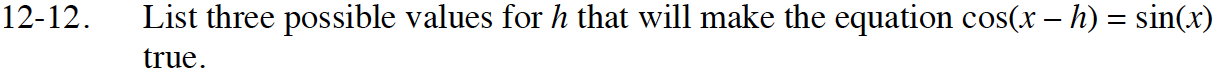### Home > INT3 > Chapter 12 > Lesson 12.1.1 > Problem12-12

12-12.

List three possible values for h that will make the equation cos(xh) = sin(x) true. Homework Help ✎How far would you need to shift the graph of cos(x) horizontally so that it is the same as sin(x)?